## How to Calculate and Solve for Total Load on Flexible Pipe | Irrigation Water Requirement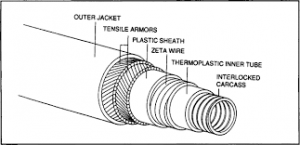The image above represents total load on flexible pipe.

To compute for total load on flexible pipe, three essential parameters are needed and these parameters are Load Co-efficient for Ditch Conduits (Cd), Unit Weight of Fill Material (w), Outside Diameter of the Conduit (Bc) and Width of Ditch at Top of Conduit (Bd).

The formula for calculating Total Load on Flexible Pipe:

Wc = CdwBcBd

Where:

Wc = Total Load on Flexible Pipe
Cd = Load Co-efficient for Ditch Conduits
w = Unit Weight of Fill Material
Bc = Outside Diameter of the Conduit
Bd = Width of Ditch at Top of Conduit

Let’s Solve an example;
Find the total load on flexible pipe when the load co-efficient for ditch conduits is 3, the unit weight of fill material is 5, the outside diameter of the conduit is 7 and width of ditch at top of conduit is 5.

This implies that;

Cd = Load Co-efficient for Ditch Conduits = 3
w = Unit Weight of Fill Material = 5
Bc = Outside Diameter of the Conduit = 7
Bd = Width of Ditch at Top of Conduit = 5

Wc = CdwBcBd
Wc = (3)(5)(7)(5)
Wc = 525

Therefore, the total load on flexible pipe is 525.

Calculating for the Load Co-efficient for Ditch Conduits when the Total Load on Flexible Pipe, the Unit Weight of Fill Material, Outside Diameter of the Conduit and the Width of Ditch at Top of Conduit is Given.

Cd = Wc / w x Bc x Bd

Where;

Cd = Load Co-efficient for Ditch Conduits
Wc = Total Load on Flexible Pipe
w = Unit Weight of Fill Material
Bc = Outside Diameter of the Conduit
Bd = Width of Ditch at Top of Conduit

Let’s Solve an example;
Find the load co-efficient for ditch conduits when the total load on flexible pipe is 40, the unit weight of fill material is 4, the outside diameter of the conduit is 6 and the Width of ditch at top of conduit is 2.

This implies that;

Wc = Total Load on Flexible Pipe = 40
w = Unit Weight of Fill Material = 4
Bc = Outside Diameter of the Conduit = 6
Bd = Width of Ditch at Top of Conduit = 2

Cd = Wc / w x Bc x Bd
Cd = 40 / 4 x 6 x 2
Cd = 40 / 48
Cd = 0.83

Therefore, the load co-efficient for ditch conduits is 0.83.

## How to Calculate and Solve for Turbine Stiffness (Correction Factor) | Irrigation Water Requirement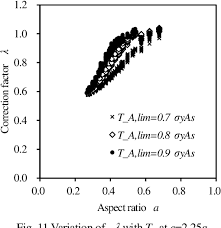The image above represents turbine stiffness.

To compute for turbine stiffness (correction factor), two essential parameters are needed and these parameters are Correction Factor for Radius of Curvature with Deflection (c) and Parallel Plate Stiffness for Tubing (σp).

The formula for calculating turbine stiffness:

σ = 0.149c[σp]

Where:

σ = Turbine Stiffness
c = Correction Factor for Radius of Curvature with Deflection
σp = Parallel Plate Stiffness for Tubing

Let’s solve an example;
Find the turbine stiffness when the correction factor for radius of curvature with deflection is 15 and the parallel plate stiffness for tubing is 3.

This implies that;

c = Correction Factor for Radius of Curvature with Deflection = 15
σp = Parallel Plate Stiffness for Tubing = 3

σ = 0.149c[σp]
σ = 0.149(15)
σ = 6.705

Therefore, the turbine stiffness is 6.705.

Calculating for the Correction Factor for Radius of Curvature with Deflection when the Turbine Stiffness and the Parallel Plate Stiffness for Tubing is Given.

c = σ / 0.149 x σp

Where;

c = Correction Factor for Radius of Curvature with Deflection
σ = Turbine Stiffness
σp = Parallel Plate Stiffness for Tubing

Let’s solve an example;
Find the correction factor for radius of curvature with deflection when the turbine stiffness is 32 and the parallel plate stiffness for tubing is 8.

This implies that;

σ = Turbine Stiffness = 32
σp = Parallel Plate Stiffness for Tubing = 8

c = σ / 0.149 x σp
c = 32 / 0.149 x 8
c = 32 / 1.192
c = 26.84

Therefore, the correction factor for radius of curvature with deflection is 26.84.

## How to Calculate and Solve for Turbine Stiffness | Irrigation Water Requirement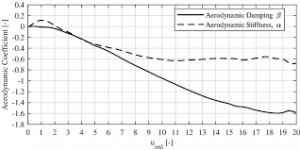The image above represents turbine stiffness.

To compute for turbine stiffness, three essential parameters are needed and these parameters are Modulus of Elasticity for Tubing (E), Moment of Inertia of Tubing (I) and Mean Radius of Tubing (r).

The formula for calculating turbine stiffness:

σ = EI /

Where:

σ = Turbine Stiffness
E = Modulus of Elasticity for Tubing
I = Moment of Inertia of Tubing
r = Mean Radius of Tubing

Let’s solve an example;
Find the turbine stiffness when the modulus of elasticity for tubing is 12, the moment of inertia of tubing is 10 and the mean radius of tubing is 4.

This implies that;

E = Modulus of Elasticity for Tubing = 12
I = Moment of Inertia of Tubing = 10
r = Mean Radius of Tubing = 4

σ = EI /
σ = (12)(10) / (4)³
σ = 120 / 64
σ = 1.875

Therefore, the turbine stiffness is 1.875.

Calculating for the Modulus of Elasticity for Tubing when the Turbine Stiffness, the Moment of Inertia of Tubing and the Mean Radius of Tubing is Given.

E = σ x r³ / I

Where;

E = Modulus of Elasticity for Tubing
σ = Turbine Stiffness
I = Moment of Inertia of Tubing
r = Mean Radius of Tubing

Let’s solve an example;
Find the modulus of elasticity for tubing when the turbine stiffness is 20, the moment of inertia of tubing is 10 and the mean radius of tubing is 2.

This implies that;

σ = Turbine Stiffness = 20
I = Moment of Inertia of Tubing = 10
r = Mean Radius of Tubing = 2

E = σ x r³ / I
E = 20 x 2³ / 10
E = 20 x 8 / 10
E = 160 / 10
E = 16

Therefore, the modulus of elasticity for tubing is 16.

## How to Calculate and Solve for Horizontal Deflection |Irrigation Water Requirement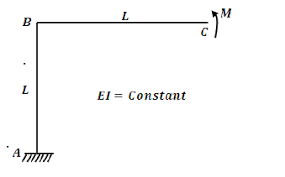The image above represents horizontal deflection.

To compute for horizontal deflection, seven essential parameters are needed and these parameters are Deflection Lag Factor (Dt), Bedding Angle Factor (K), Soil Load per Unit Length (W), Modulus of Elasticity of Tubing (E), Moment of Inertia of Tubing (I), Mean Radius of Tubing (r) and Modulus of Soil Reaction (E’).

The formula for calculating horizontal deflection:

Δx = DtKW / [EI/r³] + 0.061E’

Where:

Δx = Horizontal Deflection
Dt = Deflection Lag Factor
K = Bedding Angle Factor
W = Soil Load per Unit Length
E = Modulus of Elasticity of Tubing
I = Moment of Inertia of Tubing
r = Mean Radius of Tubing
E’ = Modulus of Soil Reaction

Let’s solve an example;
Find the horizontal deflection when the deflection lag factor is 6, the bedding angle factor is 4, the soil load per unit length is 9, the modulus of elasticity of tubing is 10, the moment of inertia of tubing is 2, the mean radius of tubing is 11 and the modulus of soil reaction is 8.

This implies that;

Dt = Deflection Lag Factor = 6
K = Bedding Angle Factor = 4
W = Soil Load per Unit Length = 9
E = Modulus of Elasticity of Tubing = 10
I = Moment of Inertia of Tubing = 2
r = Mean Radius of Tubing = 11
E’ = Modulus of Soil Reaction = 8

Δx = DtKW / [EI/r³] + 0.061E’
Δx = (6)(4)(9) / [(10)(2)/(11)³] + 0.061(8)
Δx = 216 / [20/1331] + 0.488
Δx = 216 / [0.015] + 0.488
Δx = 216 / 0.50
Δx = 429.40

Therefore, the horizontal deflection is 429.40.

## How to Calculate and Solve for Soil Load on Rigid Pipe | Irrigation Water Requirement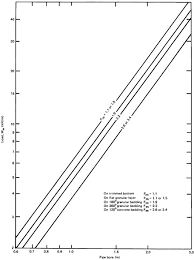The image above represents soil load on rigid pipe.

To compute for soil load on rigid pipe, three essential parameters are needed and these parameters are Load Co-efficient for Ditch Conduits (Cd), Unit Weight of Fill Material (w) and Width of Ditch at Top of Conduit (Bd).

The formula for calculating Soil Load on Rigid Pipe:

Wc = CdwBd²

Where:

Wc = Soil Load on Rigid Pipe
Cd = Load Co-efficient for Ditch Conduits
w = Unit Weight of Fill Material
Bd = Width of Ditch at Top of Conduit

Let’s Solve an example;
Find the soil load on rigid pipe when the load co-efficient for ditch conduits is 16, the unit weight of fill material is 6 and width of ditch at top of conduit is 2.

This implies that;

Cd = Load Co-efficient for Ditch Conduits = 16
w = Unit Weight of Fill Material = 6
Bd = Width of Ditch at Top of Conduit = 2

Wc = CdwBd²
Wc = (16)(6)(2)²
Wc = (16)(6)(4)
Wc = 384

Therefore, the soil load on rigid pipe is 384.

Calculating for the Load Co-efficient for Ditch Conduits when the Soil Load on Rigid Pipe, the Unit Weight of Fill Material and the Width of Ditch at Top of Conduit is Given.

Cd = Wc / w x Bd2

Where;

Cd = Load Co-efficient for Ditch Conduits
Wc = Soil Load on Rigid Pipe
w = Unit Weight of Fill Material
Bd = Width of Ditch at Top of Conduit

Let’s Solve an example;
Find the load co-efficient for ditch conduits when the soil load on rigid pipe is 40, the unit weight of fill material is 4 and the Width of ditch at top of conduit is 2.

This implies that;

Wc = Soil Load on Rigid Pipe = 40
w = Unit Weight of Fill Material = 4
Bd = Width of Ditch at Top of Conduit = 2

Cd = Wc / w x Bd2
Cd = 40 / 4 x 22
Cd = 40 / 4 x 4
Cd = 40 / 16
Cd = 2.5

Therefore, the load co-efficient for ditch conduits is 2.5.

## How to Calculate and Solve for Time Required to Irrigate | Irrigation Water Requirement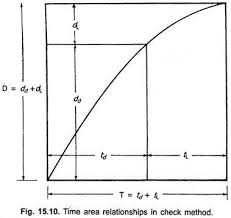The image above represents time required to irrigate.

To compute for time required to irrigate, three essential parameters are needed and these parameters are Area of the Land (A), Depth of the Water to be Applied (dwand Discharge of the Stream (q).

The formula for calculating time required to irrigate:

t = A . dw / q

Where:

t = Time Required to Irrigate
A = Area of the Land
dw = Depth of the Water to be Applied
q = Discharge of the Stream

Let’s Solve an example;
Find the time required to irrigate when the area of the land is 14, the depth of the water to be applied is 20 and the discharge of the stream is 10.

This implies that;

A = Area of the Land = 14
dw = Depth of the Water to be Applied = 20
q = Discharge of the Stream = 10

t = A . dw / q
t = 14 . 20 / 10
t = 280 / 10
t = 28

Therefore, the time required to irrigate is 28.

Calculating for the Area of the Land when the Time Required to Irrigate, the Depth of the Water to be Applied and the Discharge of the Stream is Given.

A = t x q / dw

Where;

A = Area of the Land
t = Time Required to Irrigate
dw = Depth of the Water to be Applied
q = Discharge of the Stream

Let’s Solve an example;
Find the area of the Land when the time required to irrigate is 20, the depth of the water to be applied is 12 and the discharge of the stream is 3.

This implies that;

t = Time Required to Irrigate = 20
dw = Depth of the Water to be Applied = 12
q = Discharge of the Stream = 3

A = t x q / dw
A = 20 x 3 / 12
A = 60 / 12
A = 5

Therefore, the area of the land is 5.

## How to Calculate and Solve for Depth of Available Water | Irrigation Water Requirement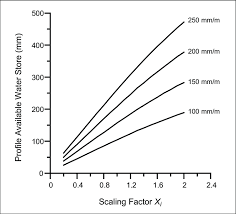The image above represents depth of available water.

To compute for depth of available water, three essential parameters are needed and these parameters are Apparent Specific Gravity of the Soil (s), Depth of the Root Zone of the Plant (d) and Water Content (m).

The formula for calculating depth of available water:

dw = s . d . m

Where:

dw = Depth of Available Water
s = Apparent Specific Gravity of the Soil
d = Depth of the Root Zone of the Plant
m = Water Content

Let’s solve an example;
Find the depth of available water when the apparent sepcific gravity of the soil is 10, the depth of the root zone of the plant is 8 and the water content is 4.

This implies that;

s = Apparent Specific Gravity of the Soil = 10
d = Depth of the Root Zone of the Plant = 8
m = Water Content = 4

dw = s . d . m
dw = (10) . (8) . (4)
dw = 320

Therefore, the depth of available water is 320.

Calculating for the Apparent Specific Gravity of the Soil when the Depth of Available Water, the Depth of the Root Zone of the Plant and the Water content is Given.

s =dw / d . m

Where;

s = Apparent Specific Gravity of the Soil
dw = Depth of Available water
d = Depth of the Root Zone of the Plant
m = Water Content

Let’s Solve an example;
Find the apparent specific gravity of the soil when the depth of available water is 60, the depth of the root zone of the plant is 10 and the water content is 3.

This implies that;

dw = Depth of Available water = 60
d = Depth of the Root Zone of the Plant = 10
m = Water Content = 3

s =dw / d . m
s =60 / 10 . 3
s =60 / 30
s = 2

Therefore, the apparent specific gravity of the soil is 2.

## How to Calculate and Solve for Capacity of the Sprinkler System | Irrigation Water Requirement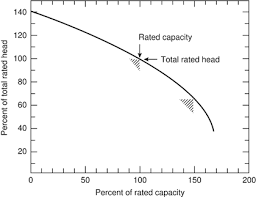The image above represents capacity of the sprinkler system.

To compute for the capacity of the sprinkler system, four essential parameters are needed and these parameters are Area to be Irrigated (A), Net Depth of Water Application (d), Number of Drop allowed for the Completion of One Irrigation (F), Number of Actual Operating Hours per Day (H) and Water Application Efficiency (E).

The formula for calculating capacity of the sprinkler system:

Q = 27800 . A . d / F . H . E

Where:

Q = Capacity of the Sprinkler System
A = Area to be Irrigated
d = Net Depth of Water Application
F = Number of Drop allowed for the Completion of One Irrigation
H = Number of Actual Operating Hours per Day
E = Water Application Efficiency

Let’s solve an example;
Find the capacity of the sprinkler system when the area to be irrigated is 2, the net depth of water application is 8, the number of drop allowed for the completion of one irrigation is 10, the number of actual operating hours per day is 6 and the water application efficiency is 4.

This implies that;

A = Area to be Irrigated = 2
d = Net Depth of Water Application = 8
F = Number of Drop allowed for the Completion of One Irrigation = 10
H = Number of Actual Operating Hours per Day = 6
E = Water Application Efficiency = 4

Q = 27800 . A . d / F . H . E
Q = 27800 . (2) . (8) / (10) . (6) . (4)
Q = 444800 / 240
Q = 1853.3

Therefore, the capacity of the sprinkler system is 1853.3.

Calculating for the Area to be Irrigated when the Capacity of the Sprinkler System, the Net Depth of Water Application, the Number of drop Allowed for the Completion of One Irrgiation, the Number of Actual Operating Hours per Day and the Water Application Efficiency is Given.

A = Q x F . H . E / 27800 . d

Where;

A = Area to be Irrigated
Q = Capacity of the Sprinkler System
d = Net Depth of Water Application
F = Number of Drop allowed for the Completion of One Irrigation
H = Number of Actual Operating Hours per Day
E = Water Application Efficiency

Let’s Solve an example;
Find the area to be irrigated when the capacity of the sprinkler system is 4, the net depth of water application is 6, number of drop allowed for the competion of one irrigation is 2, number of actual operating hours per day is 3 and the water application efficiency is 8.

This implies that;

Q = Capacity of the Sprinkler System = 4
d = Net Depth of Water Application = 6
F = Number of Drop allowed for the Completion of One Irrigation = 2
H = Number of Actual Operating Hours per Day = 3
E = Water Application Efficiency = 8

A = Q x F . H . E / 27800 . d
A = 4 x 2 . 3 . 8 / 27800 . 6
A = 192 / 166800
A = 0.00115

Therefore, the area to be irrigated is 0.00115.

## How to Calculate and Solve for Application Efficiency for Trickle Irrigation | Irrigation Water Requirement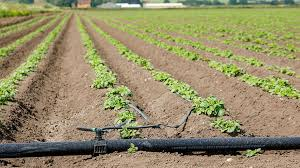The image above represents application efficiency for trickle irrigation.

To compute for application efficiency for trickle irrigation, two essential parameters are needed and these parameters are Emission Uniformity (Euand Application Efficiency for Sprinkler (Ea).

The formula for calculating application efficiency for trickle irrigation:

Eta = Eu x Ea x 100

Where:

Eta = Application Efficiency for Trickle Irrigation
Eu = Emission Uniformity
Ea = Application Efficiency for Sprinkler

Let’s solve an example;
Find the application efficiency for trickle irrigation when the emission uniformity is 32 and the applicatio efficiency for sprinkler is 4.

This implies that;

Eu = Emission Uniformity = 32
Ea = Application Efficiency for Sprinkler = 4

Eta = Eu x Ea x 100
Eta = (32) x (4) x 100
Eta = 12800

Therefore, the application efficiency for trickle irrigation is 12800%.

Calculating for the Emission Uniformity when the Application Efficiency for Trickle Irrigation and the Application Efficiency for Sprinkler is Given.

Eu = Eta / Ea x 100

Where;

Eu = Emission Uniformity
Eta = Application Efficiency for Trickle Irrigation
Ea = Application Efficiency for Sprinkler

Let’s solve an example;
Find the emission uniformity when the application efficiency for trickle irrigation is 60 and the application efficiency for sprinkler is 2.

This implies that;

Eta = Application Efficiency for Trickle Irrigation = 60
Ea = Application Efficiency for Sprinkler = 2

Eu = Eta / Ea x 100
Eu = 60 / 2 x 100
Eu = 60 / 200
Eu = 0.3

Therefore, the emission uniformity is 0.3%.

## How to Calculate and Solve for Water Distribution from Emitter | Irrigation Water Requirement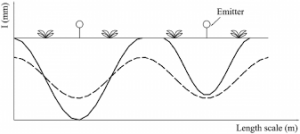The image above represents water distribution from emitter.

To compute for water distribution from emitter,two essential parameters are needed and these parameters are Manufacturer’s Co-efficient of Variation (Cvand Number of Emitters per Plant (n).

The formula for calculating water distribution from emitter:

EU = 1 – [0.8Cv / n0.5]

Where:

EU = Water Distribution from Emitter
Cv = Manufacturer’s Co-efficient of Variation
n = Number of Emitters per Plant

Let’s solve an example;
Find the water distribution from emitter when the manufacturer’s co-efficient of variation is 27 and number of emitters per plant is 3.

This implies that;

Cv = Manufacturer’s Co-efficient of Variation = 27
n = Number of Emitters per Plant = 3

EU = 1 – [0.8Cv / n0.5]
EU = 1 – [0.8(27) / (3)0.5]
EU = 1 – [21.6 / 1.732]
EU = 1 – [12.47]
EU = -11.47

Therefore, the water distribution from emitter is -11.47.

Nickzom Calculator – The Calculator Encyclopedia is capable of calculating the water distibution from emitter.

To get the answer and workings of the water distribution from emitter using the Nickzom Calculator – The Calculator Encyclopedia. First, you need to obtain the app.

You can get this app via any of these means:

To get access to the professional version via web, you need to register and subscribe for NGN 2,000 per annum to have utter access to all functionalities.
You can also try the demo version via https://www.nickzom.org/calculator

Apple (Paid) – https://itunes.apple.com/us/app/nickzom-calculator/id1331162702?mt=8
Once, you have obtained the calculator encyclopedia app, proceed to the Calculator Map, then click on Agricultural under Engineering.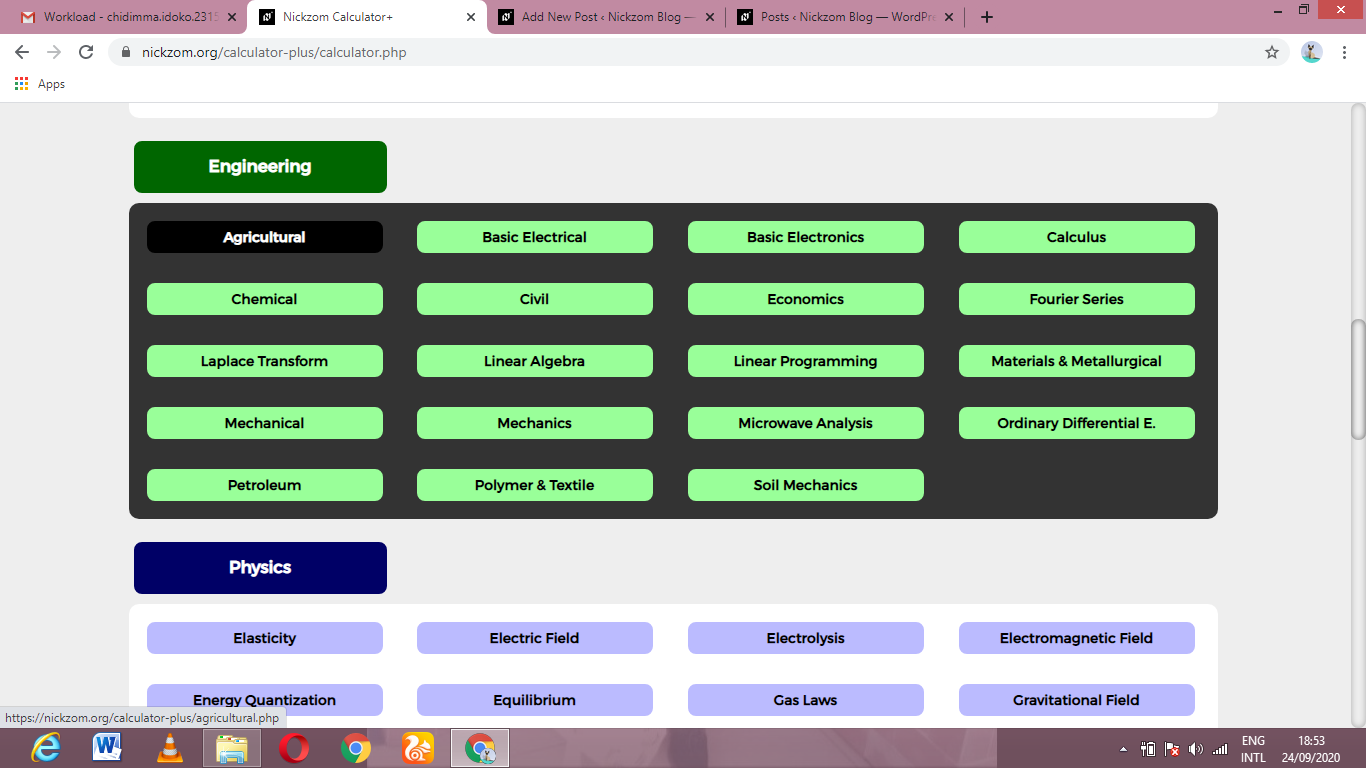Now, Click on Irrigation Water Requirement under Agricultural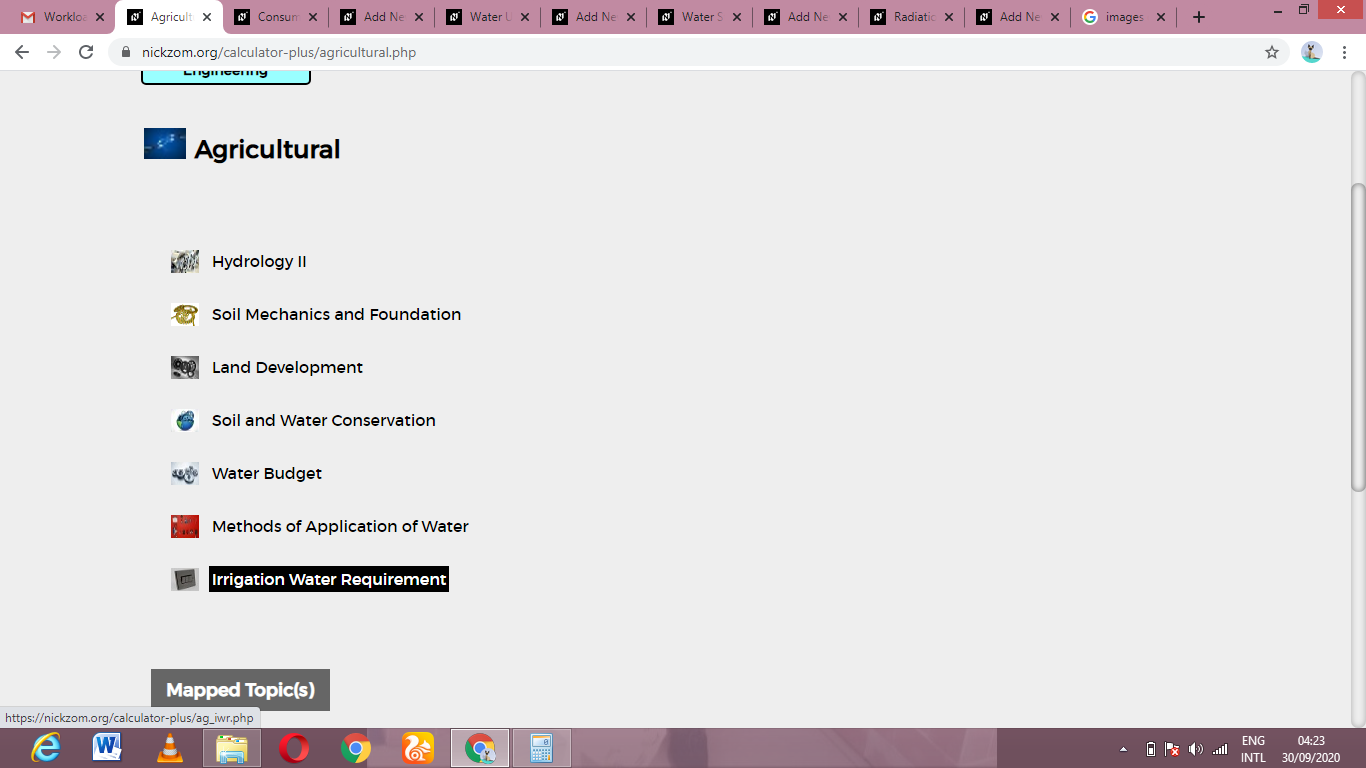Now, Click on Water Distribution from Emitter under Irrigation Water Requirement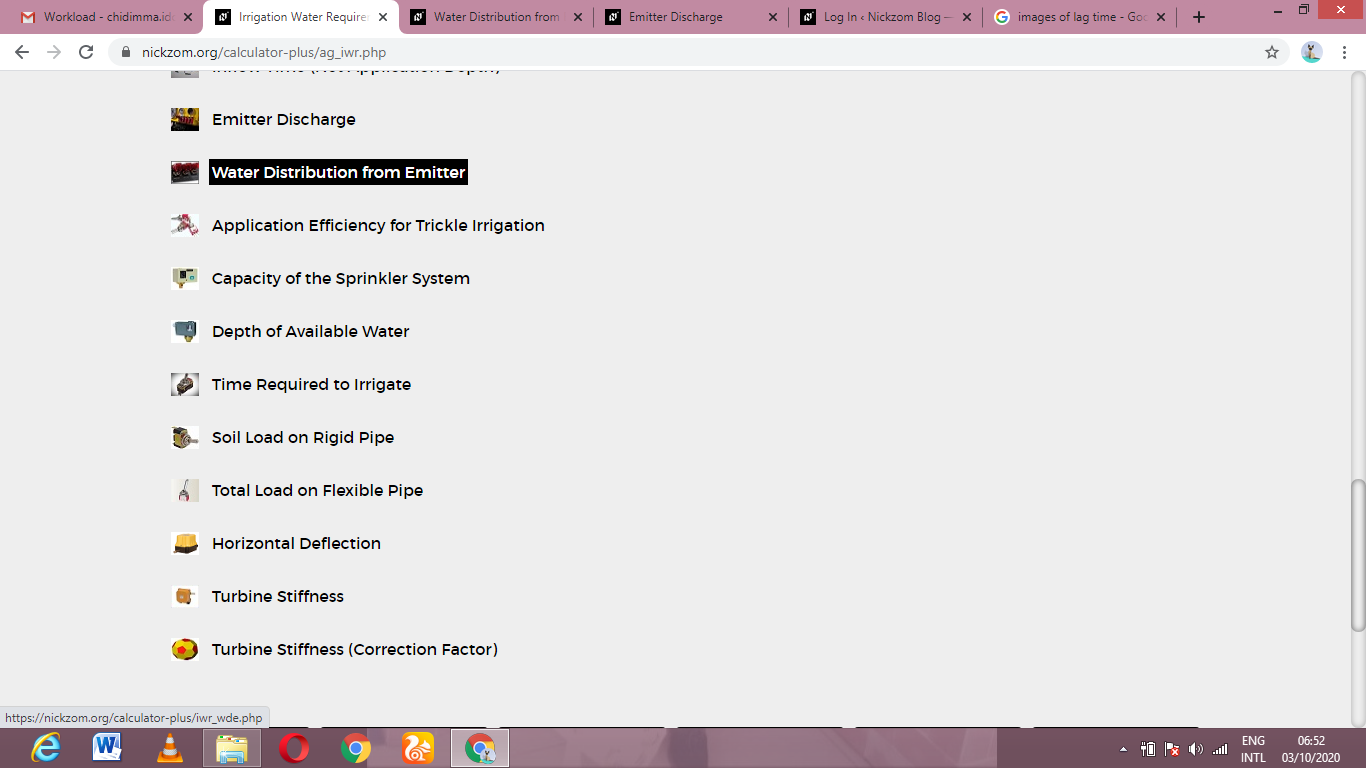The screenshot below displays the page or activity to enter your values, to get the answer for the water distribution from emitter according to the respective parameters which is the Manufacturer’s Co-efficient of Variation (Cvand Number of Emitters per Plant (n).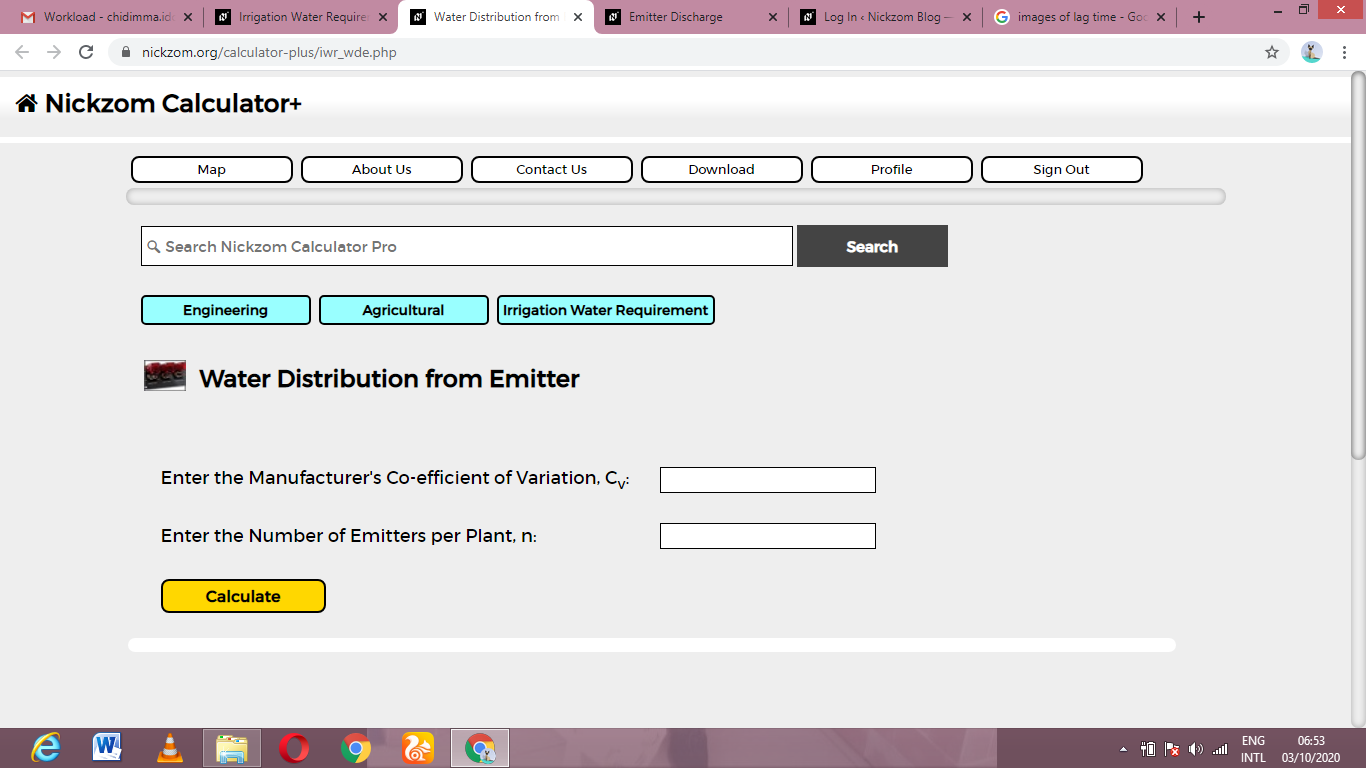Now, enter the values appropriately and accordingly for the parameters as required by the Manufacturer’s Co-efficient of Variation (Cvis 27 and Number of Emitters per Plant (n) is 3.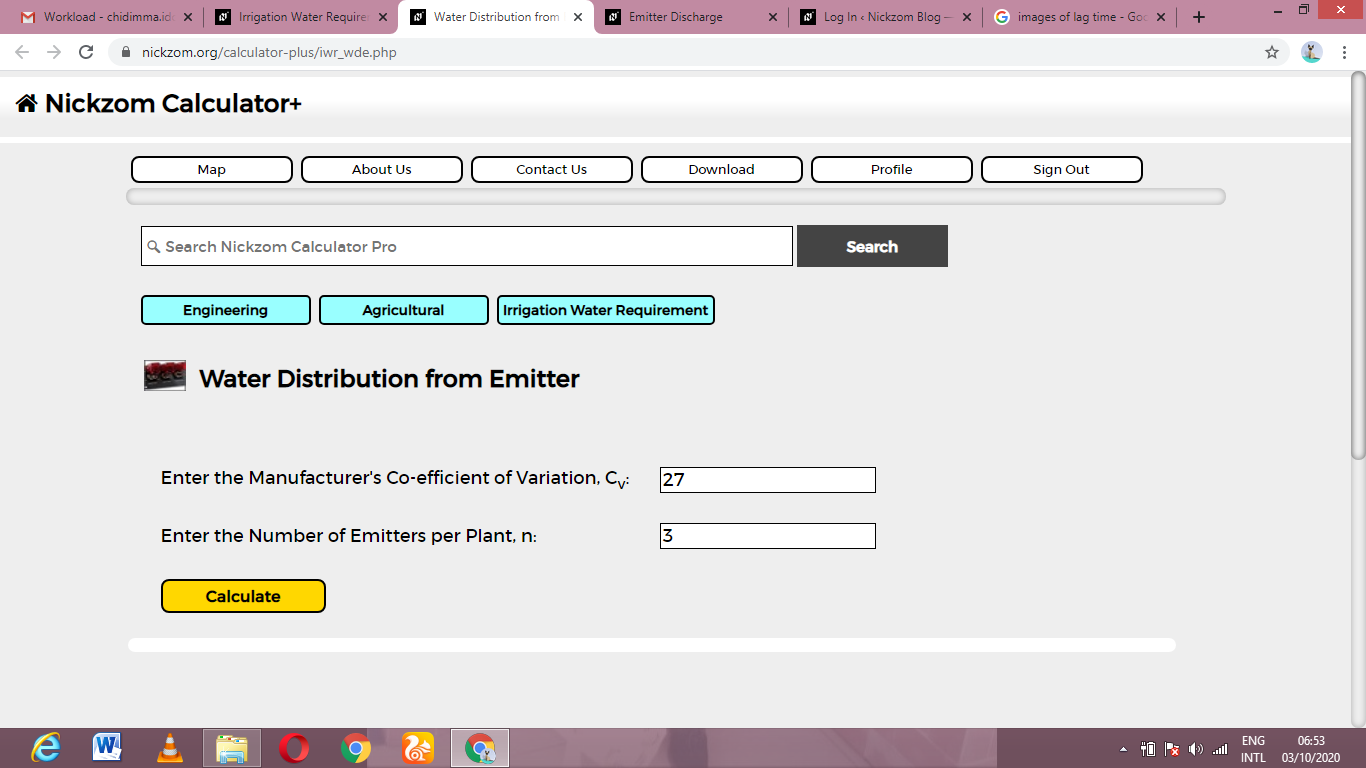Finally, Click on Calculate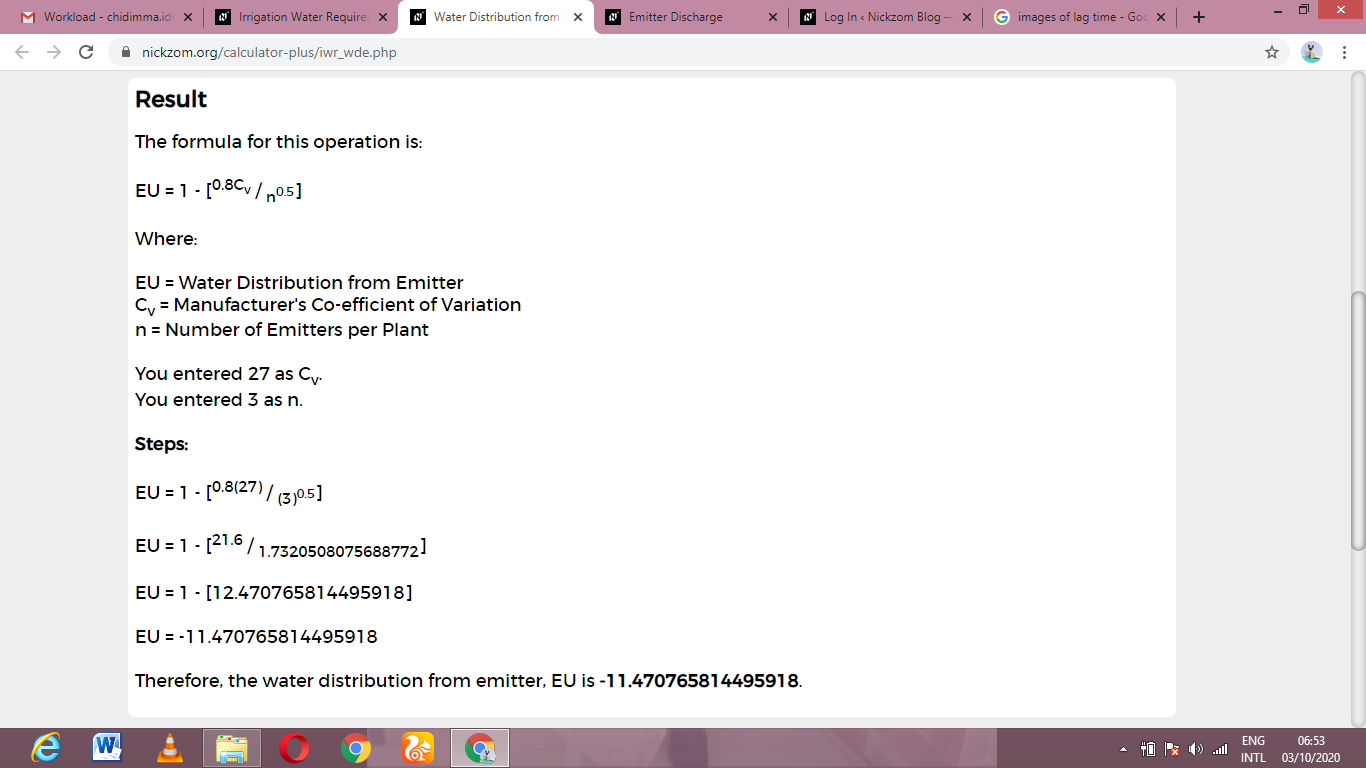As you can see from the screenshot above, Nickzom Calculator– The Calculator Encyclopedia solves for the water distribution from emitter and presents the formula, workings and steps too.